Overview
Suppose we have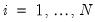independent response variables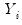, each of whose conditional mean depends on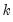-vectors of explanatory variables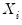and unknown coefficients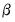. We may decomposeinto a systematic mean component,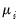, and a stochastic component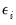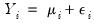(32.1)
The conventional linear regression model assumes that theis a linear predictor formed from the explanatory variables and coefficients,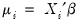, and thatis normally distributed with zero mean and constant variance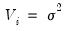.
The GLM framework of Nelder and McCullagh (1972) generalizes linear regression by allowing the mean componentto depend on a linear predictor through a nonlinear function, and the distribution of the stochastic componentbe any member of the linear exponential family. Specifically, a GLM specification consists of:
A linear predictor or index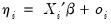where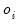is an optional offset term.
A distribution forbelonging to the linear exponential family.
A smooth, invertible link function,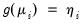, relating the meanand the linear predictor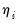.
A wide range of familiar models may be cast in the form of a GLM by choosing an appropriate distribution and link function. For example:

 Model Family Link Linear Regression Normal Identity: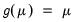Exponential Regression Normal Log: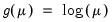Logistic Regression Binomial Logit: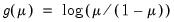Probit Regression Binomial Probit: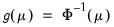Poisson Count Poisson Log:For a detailed description of these and other familiar specifications, see McCullagh and Nelder (1981) and Hardin and Hilbe (2007). It is worth noting that the GLM framework is able to nest models for continuous (normal), proportion (logistic and probit), and discrete count (Poisson) data.
Taken together, the GLM assumptions imply that the first two moments ofmay be written as functions of the linear predictor: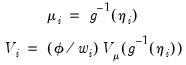(32.2)
where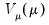is a distribution-specific variance function describing the mean-variance relationship, the dispersion constant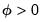is a possibly known scale factor, and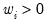is a known prior weight that corrects for unequal scaling between observations.
Crucially, the properties of the GLM maximum likelihood estimator depend only on these two moments. Thus, a GLM specification is principally a vehicle for specifying a mean and variance, where the mean is determined by the link assumption, and the mean-variance relationship is governed by the distributional assumption. In this respect, the distributional assumption of the standard GLM is overly restrictive.
Accordingly, Wedderburn (1974) shows that one need only specify a mean and variance specification as in Equation (32.2) to define a quasi-likelihood that may be used for coefficient and covariance estimation. Not surprisingly, for variance functions derived from exponential family distributions, the likelihood and quasi-likelihood functions coincide. McCullagh (1983) offers a full set of distributional results for the quasi-maximum likelihood (QML) estimator that mirror those for ordinary maximum likelihood.
QML estimators are an important tool for the analysis of GLM and related models. In particular, these estimators permit us to estimate GLM-like models involving mean-variance specifications that extend beyond those for known exponential family distributions, and to estimate models where the mean-variance specification is of exponential family form, but the observed data do not satisfy the distributional requirements (Agresti 1990, 13.2.3 offers a nice non-technical overview of QML).
Alternately, Gourioux, Monfort, and Trognon (1984) show that consistency of the GLM maximum likelihood estimator requires only correct specification of the conditional mean. Misspecification of the variance relationship does, however, lead to invalid inference, though this may be corrected using robust coefficient covariance estimation. In contrast to the QML results, the robust covariance correction does not require correction specification of a GLM conditional variance.